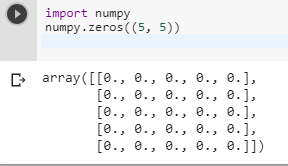2 views
in Python

I want to define a two-dimensional array without an initialized length like this:

Matrix = [][]

but it does not work...

I've tried the code below, but it is wrong too:

Matrix = 

Error:

Traceback ...

IndexError: list index out of range

What is my mistake?

by (106k points)
edited by

In Python for constructing 2D-array, you can use list-comprehension as it is the best way to construct a 2D-array. Actually in Python array is nothing but a set of list values.

# Here we are creating a list containing 5 lists, each of the 8 items, all set to 0.

a, b = 8, 5;

Matrix = [[0 for x in range(a)] for y in range(b)]

As our matrix is created, so now we can add items to it by following way:

Matrix = 1

print Matrix #it will print 1

Another way you can get a 2D-array by using numpy. Matrix operations in numpy most often used as an array type with two dimensions. There are many ways to create a new array; one of the most useful is the zeros function, which takes a shape parameter and returns an array of the given shape, with the values initialized to zero:

import numpy

numpy.zeros((5, 5))To know more about this you can have a look at the following video tutorial:-